# Interpolation (scipy.interpolate)¶

There are two general interpolation facilities available in SciPy. The first facility is an interpolation class which performs linear 1-dimensional interpolation. The second facility is based on the FORTRAN library FITPACK and provides functions for 1- and 2-dimensional (smoothed) cubic-spline interpolation.

## Linear 1-d interpolation (interp1d)¶

The interp1d class in scipy.interpolate is a convenient method to create a function based on fixed data points which can be evaluated anywhere within the domain defined by the given data using linear interpolation. An instance of this class is created by passing the 1-d vectors comprising the data. The instance of this class defines a __call__ method and can therefore by treated like a function which interpolates between known data values to obtain unknown values (it also has a docstring for help). Behavior at the boundary can be specified at instantiation time. The following example demonstrates it’s use.

>>> import numpy as np
>>> from scipy import interpolate

>>> x = np.arange(0,10)
>>> y = np.exp(-x/3.0)
>>> f = interpolate.interp1d(x, y)

>>> xnew = np.arange(0,9,0.1)
>>> import matplotlib.pyplot as plt
>>> plt.plot(x,y,'o',xnew,f(xnew),'-')

(Source code)

Output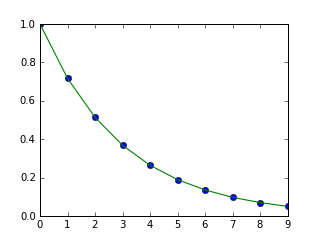(PNG, PDF)

## Spline interpolation in 1-d (interpolate.splXXX)¶

Spline interpolation requires two essential steps: (1) a spline representation of the curve is computed, and (2) the spline is evaluated at the desired points. In order to find the spline representation, there are two different was to represent a curve and obtain (smoothing) spline coefficients: directly and parametrically. The direct method finds the spline representation of a curve in a two- dimensional plane using the function splrep. The first two arguments are the only ones required, and these provide the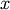and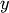components of the curve. The normal output is a 3-tuple,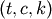, containing the knot-points,, the coefficients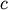and the order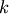of the spline. The default spline order is cubic, but this can be changed with the input keyword, k.

For curves in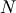-dimensional space the function splprep allows defining the curve parametrically. For this function only 1 input argument is required. This input is a list of-arrays representing the curve in-dimensional space. The length of each array is the number of curve points, and each array provides one component of the-dimensional data point. The parameter variable is given with the keword argument, u, which defaults to an equally-spaced monotonic sequence between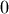and. The default output consists of two objects: a 3-tuple,, containing the spline representation and the parameter variable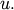The keyword argument, s , is used to specify the amount of smoothing to perform during the spline fit. The default value ofiswhere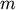is the number of data-points being fit. Therefore, if no smoothing is desired a value of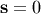should be passed to the routines.

Once the spline representation of the data has been determined, functions are available for evaluating the spline (splev) and its derivatives (splev, splade) at any point and the integral of the spline between any two points ( splint). In addition, for cubic splines (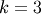) with 8 or more knots, the roots of the spline can be estimated ( sproot). These functions are demonstrated in the example that follows.

>>> import numpy as np
>>> import matplotlib.pyplot as plt
>>> from scipy import interpolate


Cubic-spline

>>> x = np.arange(0,2*np.pi+np.pi/4,2*np.pi/8)
>>> y = np.sin(x)
>>> tck = interpolate.splrep(x,y,s=0)
>>> xnew = np.arange(0,2*np.pi,np.pi/50)
>>> ynew = interpolate.splev(xnew,tck,der=0)

>>> plt.figure()
>>> plt.plot(x,y,'x',xnew,ynew,xnew,np.sin(xnew),x,y,'b')
>>> plt.legend(['Linear','Cubic Spline', 'True'])
>>> plt.axis([-0.05,6.33,-1.05,1.05])
>>> plt.title('Cubic-spline interpolation')
>>> plt.show()


Derivative of spline

>>> yder = interpolate.splev(xnew,tck,der=1)
>>> plt.figure()
>>> plt.plot(xnew,yder,xnew,np.cos(xnew),'--')
>>> plt.legend(['Cubic Spline', 'True'])
>>> plt.axis([-0.05,6.33,-1.05,1.05])
>>> plt.title('Derivative estimation from spline')
>>> plt.show()


Integral of spline

>>> def integ(x,tck,constant=-1):
>>>     x = np.atleast_1d(x)
>>>     out = np.zeros(x.shape, dtype=x.dtype)
>>>     for n in xrange(len(out)):
>>>         out[n] = interpolate.splint(0,x[n],tck)
>>>     out += constant
>>>     return out
>>>
>>> yint = integ(xnew,tck)
>>> plt.figure()
>>> plt.plot(xnew,yint,xnew,-np.cos(xnew),'--')
>>> plt.legend(['Cubic Spline', 'True'])
>>> plt.axis([-0.05,6.33,-1.05,1.05])
>>> plt.title('Integral estimation from spline')
>>> plt.show()


Roots of spline

>>> print interpolate.sproot(tck)
[ 0.      3.1416]


Parametric spline

>>> t = np.arange(0,1.1,.1)
>>> x = np.sin(2*np.pi*t)
>>> y = np.cos(2*np.pi*t)
>>> tck,u = interpolate.splprep([x,y],s=0)
>>> unew = np.arange(0,1.01,0.01)
>>> out = interpolate.splev(unew,tck)
>>> plt.figure()
>>> plt.plot(x,y,'x',out,out,np.sin(2*np.pi*unew),np.cos(2*np.pi*unew),x,y,'b')
>>> plt.legend(['Linear','Cubic Spline', 'True'])
>>> plt.axis([-1.05,1.05,-1.05,1.05])
>>> plt.title('Spline of parametrically-defined curve')
>>> plt.show()

(Source code)

Output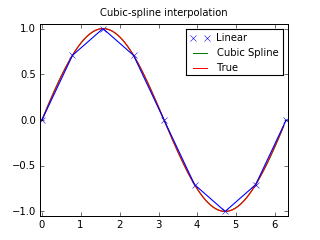(PNG, PDF)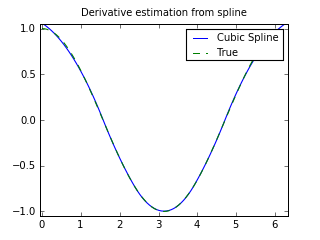(PNG, PDF)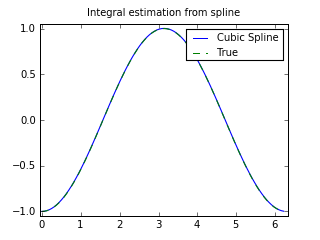(PNG, PDF)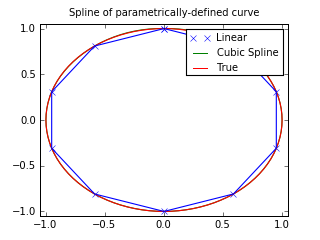(PNG, PDF)

## Two-dimensional spline representation (bisplrep)¶

For (smooth) spline-fitting to a two dimensional surface, the function bisplrep is available. This function takes as required inputs the 1-D arrays x, y, and z which represent points on the surface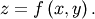The default output is a list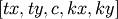whose entries represent respectively, the components of the knot positions, the coefficients of the spline, and the order of the spline in each coordinate. It is convenient to hold this list in a single object, tck, so that it can be passed easily to the function bisplev. The keyword, s , can be used to change the amount of smoothing performed on the data while determining the appropriate spline. The default value iswhereis the number of data points in the x, y, and z vectors. As a result, if no smoothing is desired, then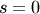should be passed to bisplrep .

To evaluate the two-dimensional spline and it’s partial derivatives (up to the order of the spline), the function bisplev is required. This function takes as the first two arguments two 1-D arrays whose cross-product specifies the domain over which to evaluate the spline. The third argument is the tck list returned from bisplrep. If desired, the fourth and fifth arguments provide the orders of the partial derivative in theanddirection respectively.

It is important to note that two dimensional interpolation should not be used to find the spline representation of images. The algorithm used is not amenable to large numbers of input points. The signal processing toolbox contains more appropriate algorithms for finding the spline representation of an image. The two dimensional interpolation commands are intended for use when interpolating a two dimensional function as shown in the example that follows. This example uses the mgrid command in SciPy which is useful for defining a “mesh-grid “in many dimensions. (See also the ogrid command if the full-mesh is not needed). The number of output arguments and the number of dimensions of each argument is determined by the number of indexing objects passed in mgrid.

>>> import numpy as np
>>> from scipy import interpolate
>>> import matplotlib.pyplot as plt


Define function over sparse 20x20 grid

>>> x,y = np.mgrid[-1:1:20j,-1:1:20j]
>>> z = (x+y)*np.exp(-6.0*(x*x+y*y))

>>> plt.figure()
>>> plt.pcolor(x,y,z)
>>> plt.colorbar()
>>> plt.title("Sparsely sampled function.")
>>> plt.show()


Interpolate function over new 70x70 grid

>>> xnew,ynew = np.mgrid[-1:1:70j,-1:1:70j]
>>> tck = interpolate.bisplrep(x,y,z,s=0)
>>> znew = interpolate.bisplev(xnew[:,0],ynew[0,:],tck)

>>> plt.figure()
>>> plt.pcolor(xnew,ynew,znew)
>>> plt.colorbar()
>>> plt.title("Interpolated function.")
>>> plt.show()

(Source code)

Output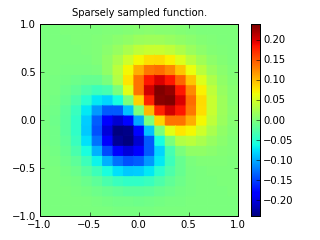(PNG, PDF)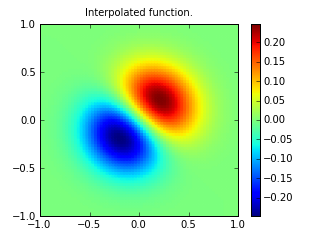(PNG, PDF)

## Using radial basis functions for smoothing/interpolation¶

Radial basis functions can be used for smoothing/interpolating scattered data in n-dimensions, but should be used with caution for extrapolation outside of the observed data range.

### 1-d Example¶

This example compares the usage of the Rbf and UnivariateSpline classes from the scipy.interpolate module.

>>> import numpy as np
>>> from scipy.interpolate import Rbf, InterpolatedUnivariateSpline
>>> import matplotlib.pyplot as plt

>>> # setup data
>>> x = np.linspace(0, 10, 9)
>>> y = np.sin(x)
>>> xi = np.linspace(0, 10, 101)

>>> # use fitpack2 method
>>> ius = InterpolatedUnivariateSpline(x, y)
>>> yi = ius(xi)

>>> plt.subplot(2, 1, 1)
>>> plt.plot(x, y, 'bo')
>>> plt.plot(xi, yi, 'g')
>>> plt.plot(xi, np.sin(xi), 'r')
>>> plt.title('Interpolation using univariate spline')

>>> # use RBF method
>>> rbf = Rbf(x, y)
>>> fi = rbf(xi)

>>> plt.subplot(2, 1, 2)
>>> plt.plot(x, y, 'bo')
>>> plt.plot(xi, yi, 'g')
>>> plt.plot(xi, np.sin(xi), 'r')
>>> plt.title('Interpolation using RBF - multiquadrics')
>>> plt.show()

(Source code)

Output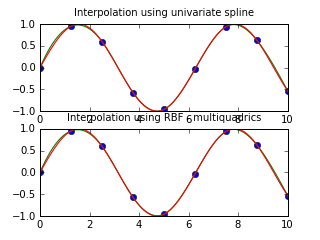(PNG, PDF)

### 2-d Example¶

This example shows how to interpolate scattered 2d data.

>>> import numpy as np
>>> from scipy.interpolate import Rbf
>>> import matplotlib.pyplot as plt
>>> from matplotlib import cm

>>> # 2-d tests - setup scattered data
>>> x = np.random.rand(100)*4.0-2.0
>>> y = np.random.rand(100)*4.0-2.0
>>> z = x*np.exp(-x**2-y**2)
>>> ti = np.linspace(-2.0, 2.0, 100)
>>> XI, YI = np.meshgrid(ti, ti)

>>> # use RBF
>>> rbf = Rbf(x, y, z, epsilon=2)
>>> ZI = rbf(XI, YI)

>>> # plot the result
>>> n = plt.normalize(-2., 2.)
>>> plt.subplot(1, 1, 1)
>>> plt.pcolor(XI, YI, ZI, cmap=cm.jet)
>>> plt.scatter(x, y, 100, z, cmap=cm.jet)
>>> plt.xlim(-2, 2)
>>> plt.ylim(-2, 2)
>>> plt.colorbar()

(Source code)

Output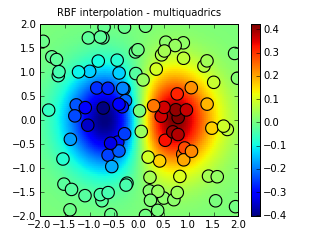(PNG, PDF)